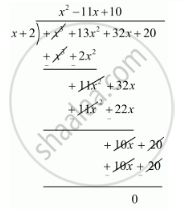# Obtain All Zeros of F(X) = X3 + 13x2 + 32x + 20, If One of Its Zeros is −2. - Mathematics

Obtain all zeros of f(x) = x3 + 13x2 + 32x + 20, if one of its zeros is −2.

#### Solution

Since −2 is one zero of f(x)

Therefore, we know that, if x = a is a zero of a polynomial, then x - a is a factor of f(x) = x + 2 is a factor of f(x)

Now, we divide f(x) = x3 + 13x2 + 32x + 20 by g(x) = (x + 2) to find the others zeros of f(x).By using that division algorithm we have,

f(x) = g(x) x q(x) + r(x)

x3 + 13x2 + 32x + 20 = (x + 2)(x2 + 11x +10) + 0

x3 + 13x2 + 32x + 20 = (x + 2)(x2 + 10x + 1x +10)

x3 + 13x2 + 32x + 20 = (x + 2)[x(x + 10) + 1(x + 10)]

x3 + 13x2 + 32x + 20 = (x + 2)(x + 1)(x + 10)

Hence, the zeros of the given polynomials are -2, -1, and -10.

Concept: Division Algorithm for Polynomials
Is there an error in this question or solution?

#### APPEARS IN

RD Sharma Class 10 Maths
Chapter 2 Polynomials
Exercise 2.3 | Q 4 | Page 57